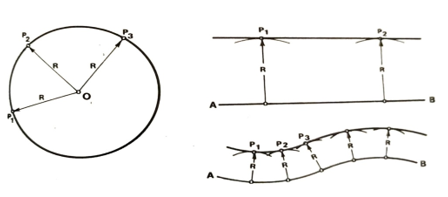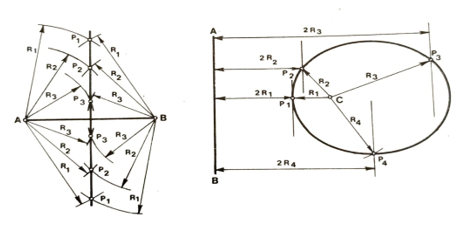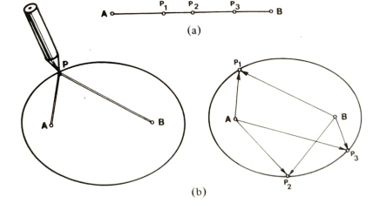# 3 Sample Loci Assignment Questions & Answers To Boost Your Knowledge

Loci is an exciting study area that deals with drawing shapes and crunching numbers. If you are struggling to master the concepts of loci, then our sample assignment questions and answers can come in handy. Our top-rated architecture assignment experts have prepared the solutions according to Ivy-League university standards. You can use the sample below to boost your knowledge of loci and test your skills. Feel free to contact us at any time if your loci assignment is giving you a hard time. We are an established provider of affordable loci assignment help. Our seasoned assignment doers are available round the clock. Procuring our service is your best chance of securing a decent grade in your loci assignment this semester.

## Question 1: Define the locus of a point

The locus of a point is the path traced by the point when it moves in accordance with specified conditions. The plural of locus is loci.

## Question 2: Which are the three common types of loci?

1. Circle
2. If a point P moves in one plane so that its distance from a fixed-point O is constant, then its locus is a circle.

To draw a circle, compasses are set to the required constant distance. With the point of the compass at O (Fig. 15.1), the compass lead then traces out the required circle through P1, P2, P3, etc., where OP=OP2 = OP3 = R, the radius of the circle.Fig. 15.1 Circle Fig                                               15.2 Parallel line

3. Parallel line
4. If a point P moves in one plane so that its perpendicular distance from a fixed line AB is constant, then its locus is a line parallel to AB.

To draw a parallel line (Fig. 15.2):

With centres on AB, strike several arcs of radius R equal to To draw a parallel line

Draw a common tangent to all these arcs. This is the required distance between AB and the parallel line. parallel line.

5. Perpendicular line
6. If a point P moves in one plane so that it is equidistant from two fixed points A and B, then its locus is a straight line perpendicular to AB.

To draw a perpendicular line (Fig. 15.3):

With centres at A and B, strike two arcs each of an arbitrary radius R, to intersect at P, on either side of AB.

2 With the same centres, strike further pairs of arcs with radii R2, R3,..., etc. and intersection points P2, P3.... on either side of AB. A straight line drawn through P1, P2, P3,..., etc. is the required line perpendicular to AB (and is also the bisector of AB).Fig. 15.3 Perpendicular line                                     Fig. 15.4 Ellipse

## Question 3: Explain in detail two methods of drawing an ellipse

Ellipse - method 1

If a point P moves in one plane so that its distance from a fixed point C and its perpendicular distance from a fixed line AB is always in the same ratio 1:n, where n is any number greater than 1, then the locus of the point is an ellipse.

To draw an ellipse (Fig. 15.4):

1. Taking a distance ratio of, say, 1:2 draw a line parallel to AB and at an arbitrary distance 2R, from it.
2. From centre C, strike an arc of radius R, to intersect this line at P₁.
3. Repeat steps 1 and 2 with radii R2, R3,..., etc. to give intersection points P2, P3,..., etc.
4. Join all the intersection points by a smooth curve. This curve is the required ellipse.

Ellipse-method 2

If a point P moves in one plane so that the sum of its distances from two fixed points A and B is constant, then its locus is again an ellipse. If a piece of string of total length equal to AP+ PB is fixed with its ends at A and B and is kept taut by a pencil held against it inside the loop so formed, moving the pencil will produce a locus which is an ellipse.

To draw an ellipse (Fig. 15.5):

1. For measuring purposes, draw a construction line of the total length equal to AP+PB (Fig. 15.5(a)).
2. Draw the two fixed points A and B (Fig. 15.5(b)).
3. From centre A, strike an arc with radius AP₁.
4. From the centre, B, strike an arc with a radius BP, measured from the construction line. This arc intersects the first one at P₁.
5. Repeat steps 3 and 4 with various distances and intersection points P2, P3,..., etc. 6 Join all the intersection points by a smooth curve. The resulting locus is an ellipse.Fig. 15.5 Ellipse